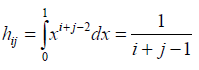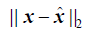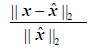On Regularisation and Comparison of Methods for Solving Hilbert Linear Systems | OMICS International
Journal of Physical Mathematics

# On Regularisation and Comparison of Methods for Solving Hilbert Linear Systems

Azizu S*

Faculty of Mathematical Sciences, Mathematics Department, Navrongo Campus, Ghana

Corresponding Author:
Azizu S
Faculty of Mathematical Sciences
Mathematics Department, Navrongo Campus
Ghana
Tel: +23304113473
E-mail: [email protected]

Received date: March 31, 2016; Accepted date:April 29, 2016; Published date: May 05, 2016

Citation: Azizu S (2016) On Regularisation and Comparison of Methods for Solving Hilbert Linear Systems. J Phys Math 7: 173. doi:10.4172/2090-0902.1000173

Copyright: © 2016 Azizu S. This is an open-access article distributed under the terms of the Creative Commons Attribution License, which permits unrestricted use, distribution, and reproduction in any medium, provided the original author and source are credited.

Visit for more related articles at Journal of Physical Mathematics

#### Abstract

This paper deals with linear ill-posed problems relating to Hilbert Systems using comparisons of methods (Matlab Backslash, SVD, GMRES, QR, and PCG) including their regularizations. Hilbert systems of varying sizes were investigated and several results on these methods were presented in tabular form based on error and relative error norm. From our numerical results ran on Matlab version 7.01 only QR regularization method is recommended for the solution of Hilbert linear system.

#### Keywords

Hilbert system; SVD decomposition; GMRES; QR factorisation; Error norm

#### Introduction

Recently, structured perturbations for linear systems have attracted much attention [1-3]. When solving linear systems of equations, it is important to analyze  how small perturbations of the matrix and right-hand side affect the solution. It is widely known that the solutions of linear systems of equations are sensitive  to round-off error and always ill-posed  to solve a linear system with Hilbert coefficient matrix due to its large condition number. Therefore, stable and efficient algorithms are needed to reduce the ill-posedness and get effective solutions for such kinds of Hilbert matrix equations. It is well known that for a system of equations with an ill-conditioned matrix, an erroneous solution can be obtained which seems to satisfy the system quite well. Various measures  of the ill-conditioning of a matrix have been proposed.

#### Related Works

The Generalized Minimal Residual (GMRES) method computes a sequence of orthogonal vectors with least-squares approach . The Gmres method combines with preconditioning in the solutions of linear systems in order to speed up convergence. This method is useful for general nonsymmetric matrices and the most popular Krylov subspace method applicable to any invertible matrix A. The roster of standard matrix decompositions  includes the pivoted QR factorization, the eigenvalue decomposition, and the singular value decomposition (SVD), all of which expose the numerical range of a matrix. SVD and truncated SVD (TSVD) methods were utilized for solving discrete illposed problems .

#### Regularization Method

The standard method to solve ill-conditioned systems known as Regularization has been studied . Regularization methods use known information about the solution for solving ill-conditioned systems. The problem is highly sensitive to small perturbation in the sense that small perturbation in the data cause large changes in the solution.

Singular value decomposition

SVD is very powerful and useful matrix decomposition, particularly in the context of data analysis, reducing transformations of images, and satellite data and is the method of choice for solving most linear least– squares problems. The SVD is intimately related to the familiar theory of diagonalizing a symmetric matrix. The SVD  is an extension of the diagonalization of a matrix. The diagonalization of a matrix is applicable only to square matrices and only to those that satisfy a quite demanding condition. SVD is a classical method  for extracting feature vectors in data.

QR factorisation

The QR decomposition (also called the QR factorization) of a matrix is a decomposition of the matrix into an orthogonal matrix and a triangular matrix. A QR decomposition of a real square matrix A is a decomposition of A as A=QR. The QR decomposition is valid for rectangular matrices as well square ones. This decomposition can be used for solving n × n linear systems but is also useful in solving overdetermined systems such as those in linear least squares. The decomposition will be used in a general algorithm for finding all eigenvalues and eigenvectors of a matrix. The two approaches , matrix and vector equations in QR factorization are powerful general tools and appeared to be applicable to the perturbation analysis of any matrix factorization.

Matlab backslash

To emphasize the distinction between solving linear equations and computing in- verses, Matlab has introduced nonstandard notation  using backward slash operator, “\” If A is a matrix of any size and shape and b (the right-hand side vector) is a matrix with as many rows as A, then the matlab backlash can be used to solved the solution vector x in Ax=B.

Generalized minimal residual (GMRES)

The Generalized Minimal Residual (GMRES) method computes a sequence of orthogonal vectors and combines these through leastsquares method. This method combines with preconditioning method to speed up convergence. However, CG requires storing the whole sequence, so that a large amount of storage is needed. For this reason, restarted versions of this method are used. GMRES is the most popular Krylov subspace method applicable to any invertible matrix A.

The performance of the conjugate gradient  method improves when the eigenvalues of matrix A are clustered about a point. This suggests the possibility of preconditioning A by a positive definite matrix M and solving M-1Ax=M-1b. If the eigenvalues of M-1A were clustered the conjugate gradient procedure may con verge at a faster rate. The preconditioned M, should be chosen to minimize the solution time. There are_ however competing priorities. Thus for example the optimal choice of M as far as clustering eigenvalues is concerned is M=A. This choice requires a direct solution of the original system and thus has an extreme cost.

#### Numerical Experiments and Results

In this paper, we present some numerical results to show the performance of error and relative error=of ill-conditioned linear systems. We ran our algorithm using MATLAB software version 7.0.1 on Intel(R) Pentium (R) CPU P600 @ 1.87GHz 1.87 and Installed Memory (RAM): 4.00GB. With regard to Hilbert system of linear equations using the MATLAB command for the coefficient matrix A and RHS vector b where the exact solution is x=ones (n,1) for the discussion to test for illconditioning of the system, varying sizes(n) of the Hilbert matrix were considered as shown in the Tables 1-5. We started with n=10, n=20, n=30, n=60 and n=120 and in each computation, the matlab backslash, QR, SVD,GMRES, PCG and their regularizations (RMatlab (“\”), RQR RSVD,RGMRES, RPCG) were used to determine the error and relative error of each size of Hilbert matrix. The Hilbert matrix HòRn×n with entriesMethodsMatlab(“\”) 7.8918e-004 2.4956e-004
QR 6.5264 2.0638
SVD 1.7180e+013 5.4329e+012
GMRES 0.0345 0.0109
PCG 3.1623 1.0000
Regularization(R)
R Matlab(“\”) 8.9523 2.8310
RQR 0.2853 0.0902
R SVD 6.6621e+003 2.1068e+003
R GMRES 0.2856 0.0903
R PCG 0.2856 0.0903

Table 1: Hilbert System n=10.

MethodsMatlab(“\”) 66.2923 14.8234
QR 9.1309 2.0417
SVD 1.7847e+018 3.9906e+017
GMRES 0.0325 0.0073
PCG 4.4721 1.0000
Regularization (R)
R Matlab(“\”) 191.6066 42.8445
RQR 0.3890 0.0870
R SVD 1.1806e+004 2.6400e+003
R GMRES 0.3890 0.0870
R PCG 0.3890 0.0870

Table 2: Hilbert System n=20.

MethodsMatlab(“\”) 106.9835 19.5324
QR 11.1001 2.0266
SVD 2.7502e+018 5.0212e+017
GMRES 0.0203 0.0037
PCG 5.4772 1.0000
Regularization (R)
R Matlab(“\”) 7.5318e+003 1.3751e+003
RQR 0.5102 0.0932
R SVD 1.5385e+004 2.8090e+003
R GMRES 0.5103 0.0932
R PCG 0.5103 0.0932

Table 3: Hilbert System n=30.

MethodsMatlab(“\”) 212.1060 27.3828
QR 15.4956 2.0005
SVD 1.0164e+019 1.3121e+018
GMRES 0.0628 0.0081
PCG 7.7460 1.0000
Regularization (R)
R Matlab(“\”) 1.4314e+003 184.7867
RQR 0.7052 0.0910
R SVD 2.4649e+004 3.1821e+003
R GMRES 0.7090 0.0915
R PCG 0.7090 0.0915

Table 4: Hilbert System n=60.

MethodsMatlab(“\”) 5.3477e+003 488.1792
QR 21.1229 1.9282
SVD 4.3154e+019 3.9394e+018
GMRES 0.0665 0.0061
PCG 10.9545 1.0000
Regularization (R)
RMatlab(“\”) 3.0006e+003 273.9158
R QR 0.9843 0.0899
R SVD 3.0943e+004 2.8247e+003
R GMRES 0.9843 0.0899
R PCG 0.9843 0.0899

Table 5: Hilbert System n=120.

#### Discussions

The tables of the numerical experiments of the Hilbert linear system using varying sizes showed the error and relative error norm of each identified methods. Generally, the error and relative error norms of the regularizations of GMRES are equal in value. Again, from Table 1 the error and relative error norm of regularization of QR factorization has improved the Hilbert linear systems as compared to the other identified methods. Finally, only QR regularization method is recommended for the solution of Hilbert linear system.

#### Conclusion and Future Work

The regularization methods of Hilbert linear systems has been presented using varying sizes to compare the performance of the identified methods in terms of error and relative error norm. The regularization methods of Matlab, GMRES, SVD and PCG did not improve the reduction of the error and relative error norm of the identified system. We therefore recommended the regularized QR factorization method when solving Hilbert linear system.

Future work to be considered is Vander monde linear system and compares it with the known Krylov sub space methods GMRES and PCG.

#### References

Select your language of interest to view the total content in your interested language

### Article Usage

• Total views: 9362
• [From(publication date):
June-2016 - Feb 28, 2020]
• Breakdown by view type
• HTML page views : 9177Can't read the image? click here to refresh